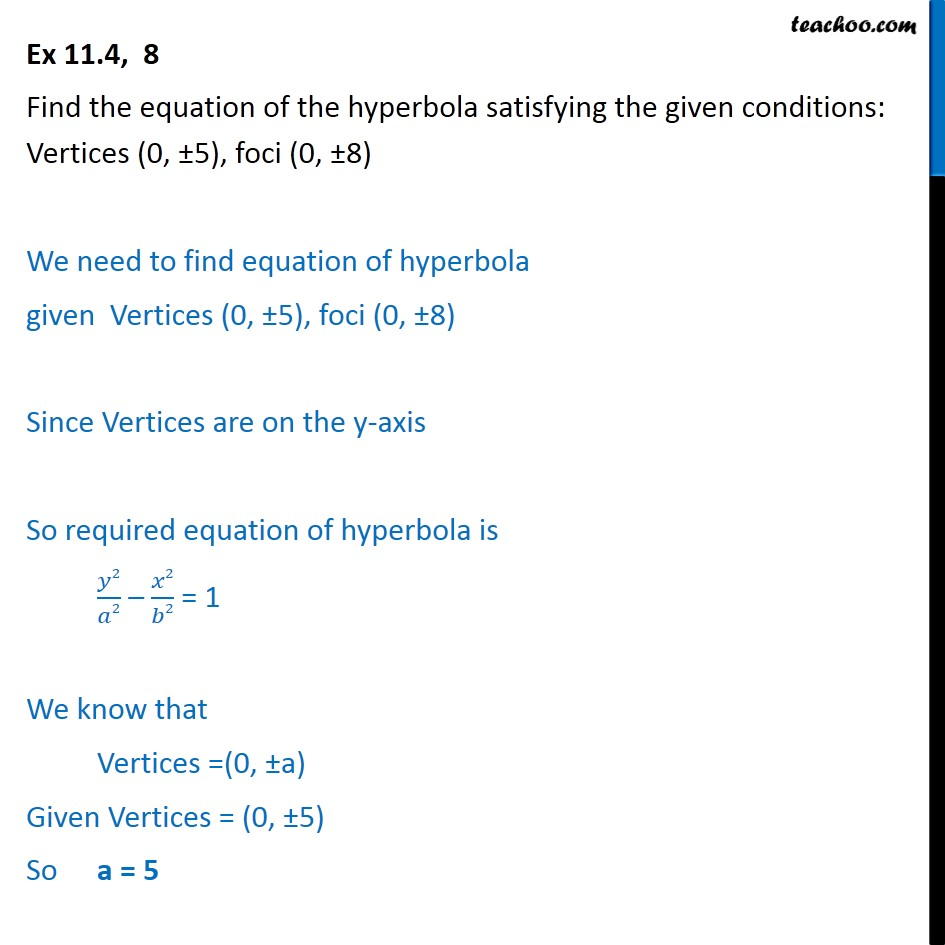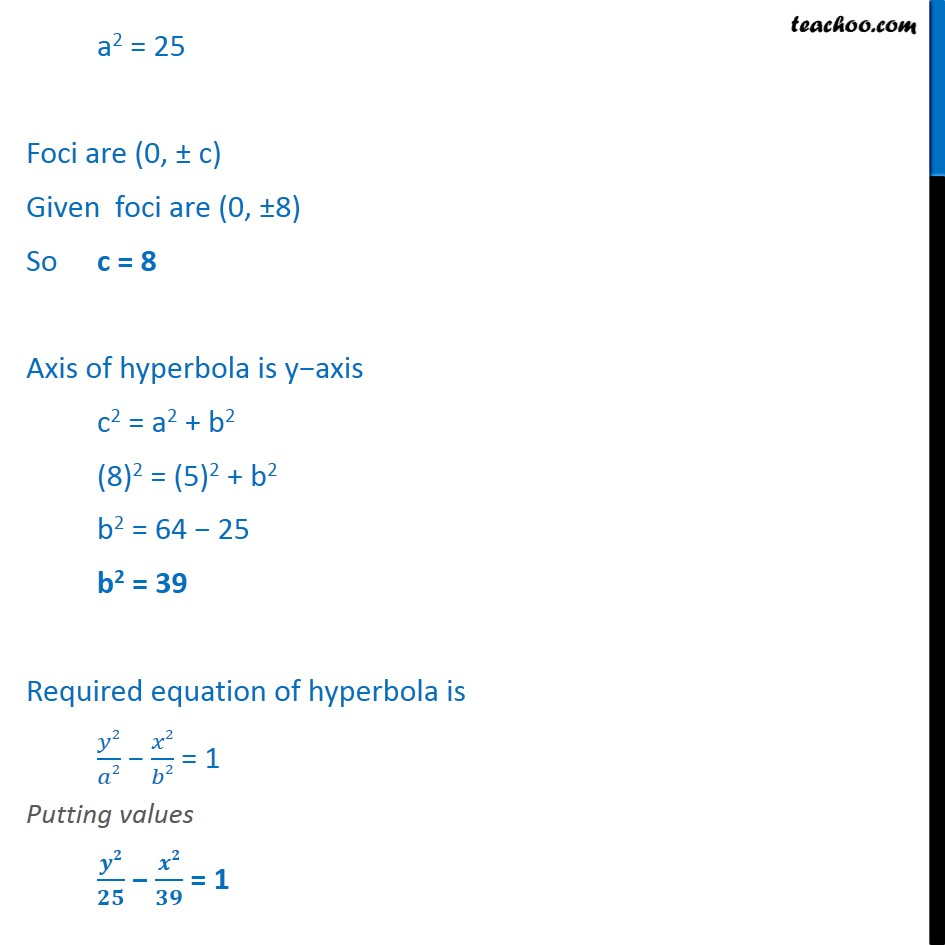Ex 10.4

Chapter 10 Class 11 Conic Sections
Serial order wiseLearn in your speed, with individual attention - Teachoo Maths 1-on-1 Class

### Transcript

Ex 10.4, 8 Find the equation of the hyperbola satisfying the given conditions: Vertices (0, 5), foci (0, 8) We need to find equation of hyperbola given Vertices (0, 5), foci (0, 8) Since Vertices are on the y-axis So required equation of hyperbola is 2 2 2 2 = 1 We know that Vertices =(0, a) Given Vertices = (0, 5) So a = 5 a2 = 25 Foci are (0, c) Given foci are (0, 8) So c = 8 Axis of hyperbola is y axis c2 = a2 + b2 (8)2 = (5)2 + b2 b2 = 64 25 b2 = 39 Required equation of hyperbola is 2 2 2 2 = 1 Putting values = 1